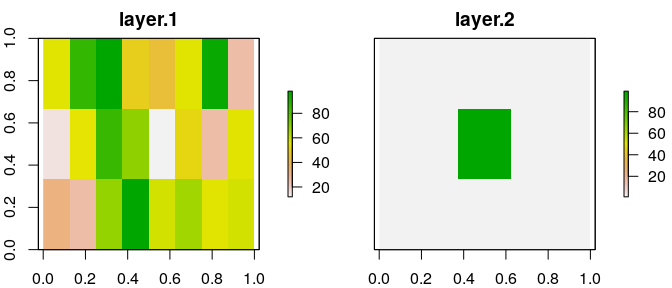# belg

Boltzmann entropy (also called configurational entropy) has been recently adopted to analyze entropy of landscape gradients (Gao et al. (2017), Gao et al. (2018)). The goal of belg is to provide an efficient C++ implementation of this method in R. It also extend the original idea by allowing calculations on data with negative and missing values.

## Installation

You can install the released version of belg from CRAN with:

``install.packages("belg")``

And the development version from GitHub with:

``````# install.packages("devtools")

## Examples

As an example, we use two small rasters - `complex_land` representing a complex landscape and `simple_land` representing a simple landscape:

``````library(raster)
library(belg)
plot(stack(complex_land, simple_land))``````The main function in this package, `get_boltzmann`, calculates the Boltzmann entropy of a landscape gradient:

``````get_boltzmann(complex_land)
#>  48.43241
get_boltzmann(simple_land)
#>  18.3818``````

This function accepts a `RasterLayer`, `RasterStack`, `RasterBrick`, `matrix`, or `array` object as an input. It also allows for calculation of the relative (the `relative` argument equal to `TRUE`) and absolute Boltzmann entropy of a landscape gradient. As a default, it uses a logarithm of base 10 (`log10`), however `log` and `log2` are also available options for the `base` argument.

``````get_boltzmann(complex_land, base = "log")
#>  111.5198
get_boltzmann(complex_land, relative = TRUE)
#>  35.50168
get_boltzmann(complex_land, base = "log2", relative = TRUE)
#>  117.934``````
• Gao, Peichao, Hong Zhang, and Zhilin Li. “An efficient analytical method for computing the Boltzmann entropy of a landscape gradient.” Transactions in GIS (2018).
• Gao, Peichao, Hong Zhang, and Zhilin Li. “A hierarchy-based solution to calculate the configurational entropy of landscape gradients.” Landscape Ecology 32(6) (2017): 1133-1146.